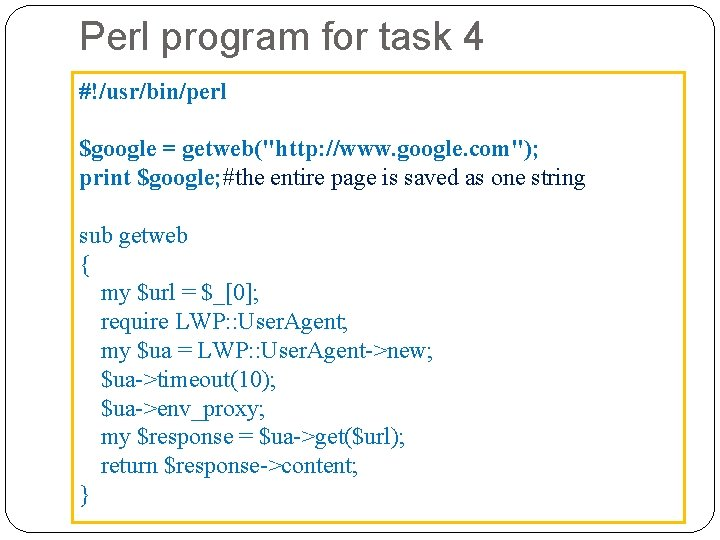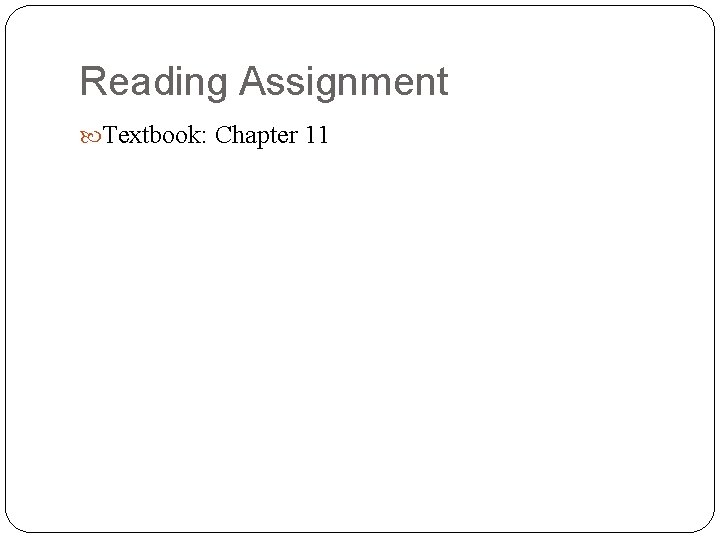# Introduction to Perl What is Perl is an

• Slides: 46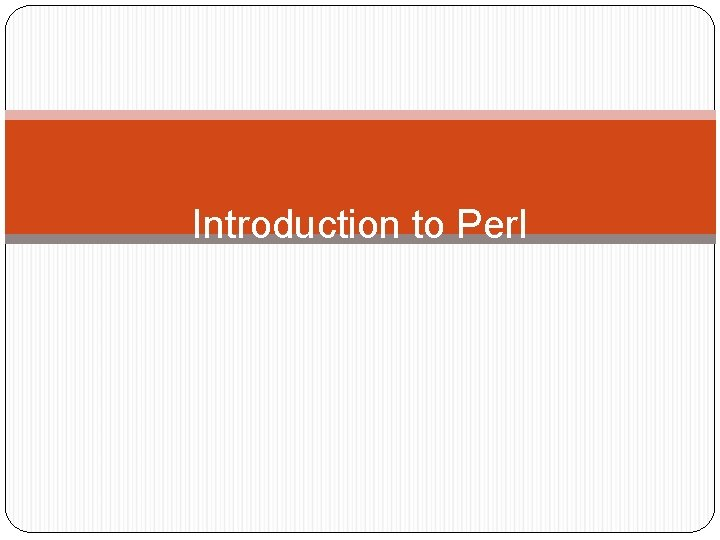Introduction to Perl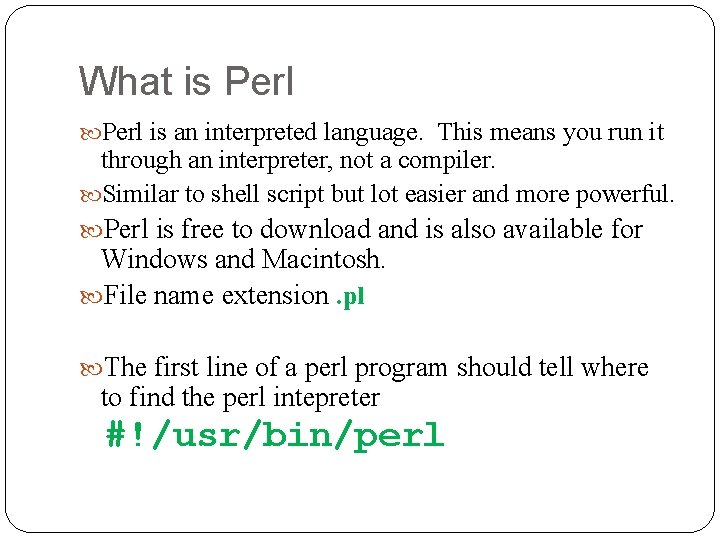What is Perl is an interpreted language. This means you run it through an interpreter, not a compiler. Similar to shell script but lot easier and more powerful. Perl is free to download and is also available for Windows and Macintosh. File name extension. pl The first line of a perl program should tell where to find the perl intepreter #!/usr/bin/perl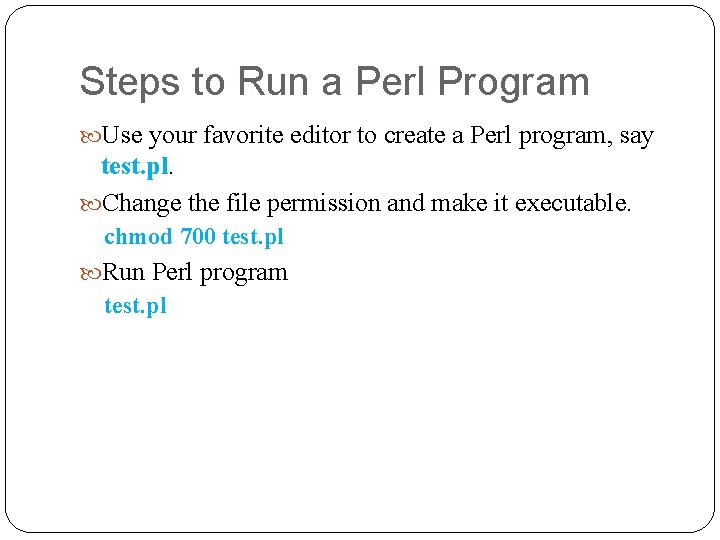Steps to Run a Perl Program Use your favorite editor to create a Perl program, say test. pl. Change the file permission and make it executable. chmod 700 test. pl Run Perl program test. pl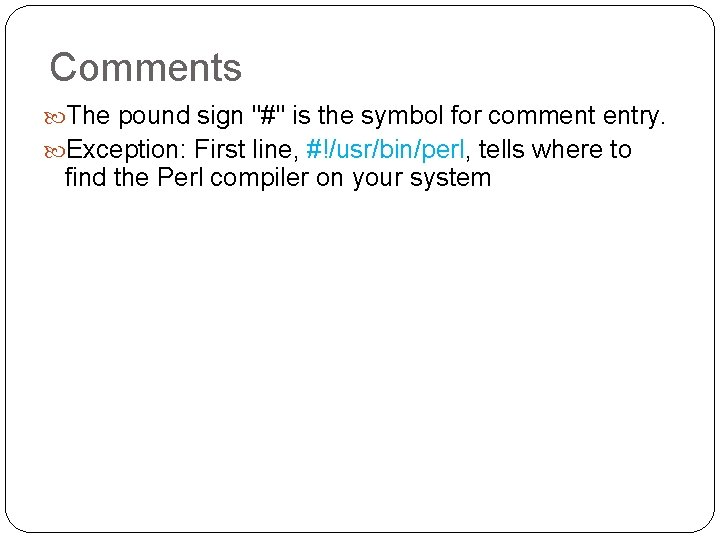Comments The pound sign "#" is the symbol for comment entry. Exception: First line, #!/usr/bin/perl, tells where to find the Perl compiler on your system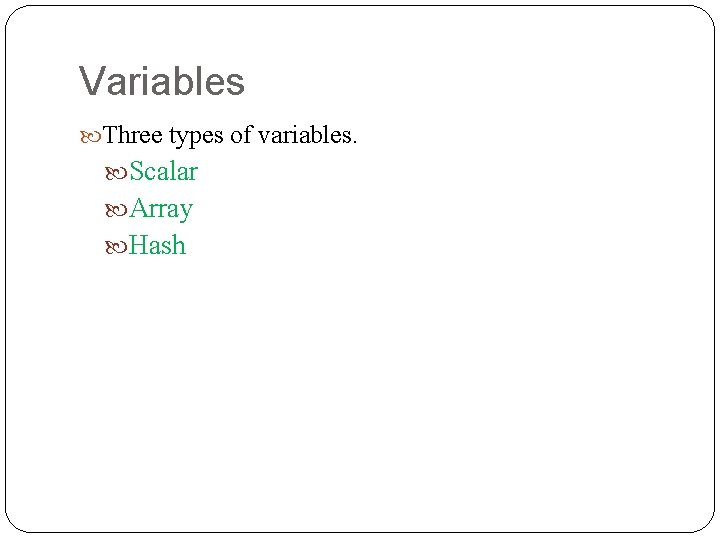Variables Three types of variables. Scalar Array Hash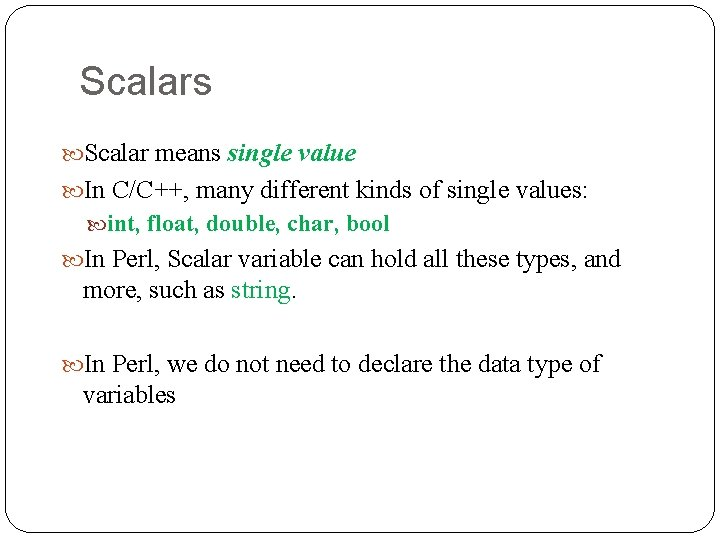Scalars Scalar means single value In C/C++, many different kinds of single values: int, float, double, char, bool In Perl, Scalar variable can hold all these types, and more, such as string. In Perl, we do not need to declare the data type of variables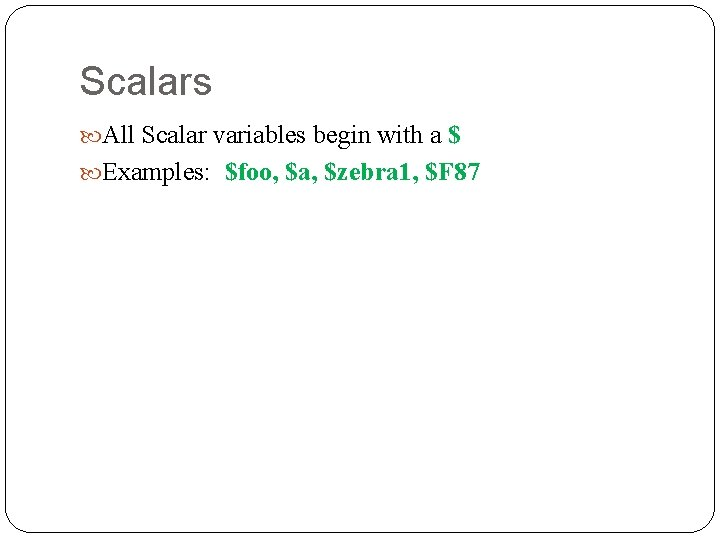Scalars All Scalar variables begin with a \$ Examples: \$foo, \$a, \$zebra 1, \$F 87Scalar Assignments Scalars hold any data type: \$foo = 3; \$d = 4. 43; \$temp = ‘Z’; #could be double quote \$My_String = “Hello, I’m Paul. ” # could be single quote \$value = TRUE;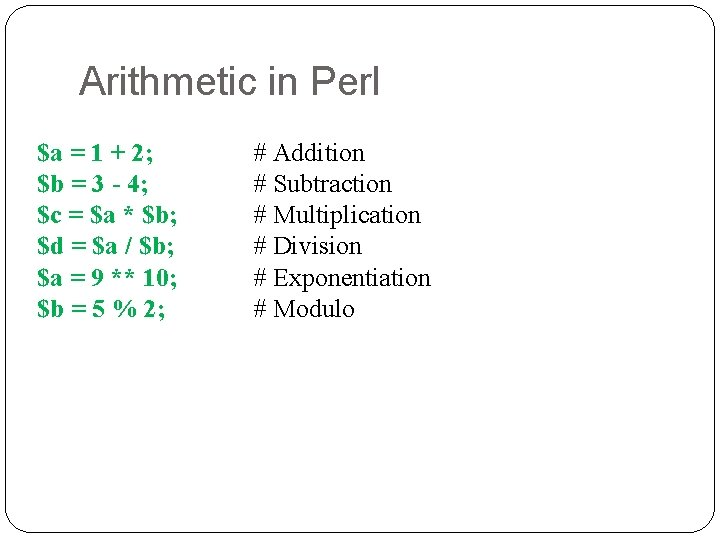Arithmetic in Perl \$a = 1 + 2; \$b = 3 - 4; \$c = \$a * \$b; \$d = \$a / \$b; \$a = 9 ** 10; \$b = 5 % 2; 9 # Addition # Subtraction # Multiplication # Division # Exponentiation # Modulo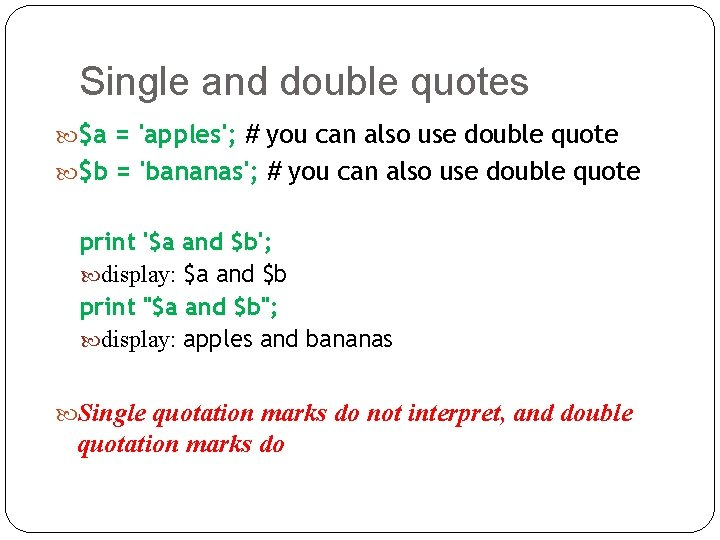Single and double quotes \$a = 'apples'; # you can also use double quote \$b = 'bananas'; # you can also use double quote print '\$a and \$b'; display: \$a and \$b print "\$a and \$b"; display: apples and bananas Single quotation marks do not interpret, and double quotation marks do 10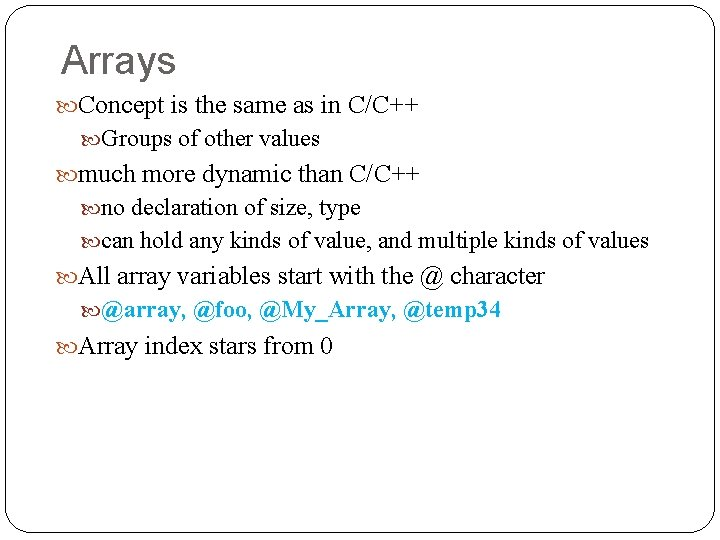Arrays Concept is the same as in C/C++ Groups of other values much more dynamic than C/C++ no declaration of size, type can hold any kinds of value, and multiple kinds of values All array variables start with the @ character @array, @foo, @My_Array, @temp 34 Array index stars from 0Array Operations @a 1 = (3, 2, 1, 4); @a 2 = (34, ‘z’, “Hi”, 43. 2); Assignment \$a 2 = ‘X’; #@a 2: (34, ‘z’, ‘X’, 43. 2) Copy array @a 3 = @a 1; #@a 3: (3, 2, 1, 4); Merge array: @a 5 = (@a 1, @a 2); #@a 5: (3, 2, 1, 4, 34, ‘z’, “Hi”, 43. 2) How about this operation: @a 1 = (@a 1, @a 2); #@a 1: (3, 2, 1, 4, 34, ‘z’, “Hi”, 43. 2)Sort Array @a 1 = (3, 2, 1, 4); Sort array @a 4 = sort (@a 1) #@a 4: (1, 2, 3, 4) How about this one? @a 1 = (3, 22, 11, 4); @a 4 = sort (@a 1) #@a 4: (11, 22, 3, 4) This is same as @a 4 = sort {\$a cmp \$b }(@a 1); Array is sorted alphabetically (elements are considered as string)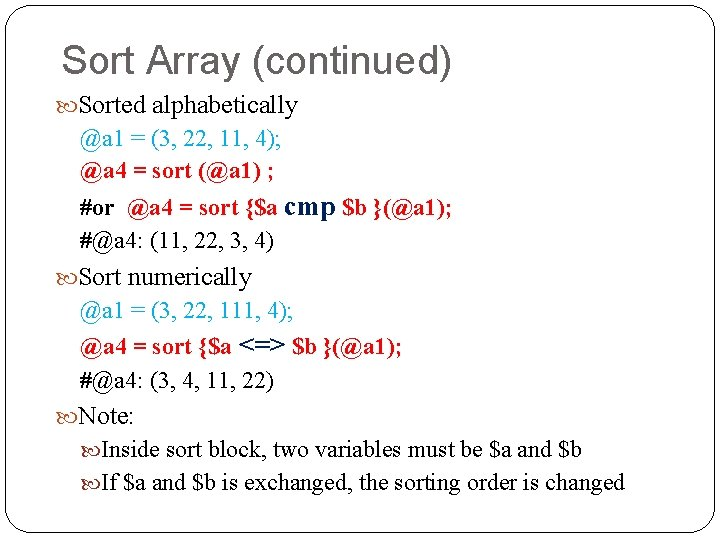Sort Array (continued) Sorted alphabetically @a 1 = (3, 22, 11, 4); @a 4 = sort (@a 1) ; #or @a 4 = sort {\$a cmp \$b }(@a 1); #@a 4: (11, 22, 3, 4) Sort numerically @a 1 = (3, 22, 111, 4); @a 4 = sort {\$a <=> \$b }(@a 1); #@a 4: (3, 4, 11, 22) Note: Inside sort block, two variables must be \$a and \$b If \$a and \$b is exchanged, the sorting order is changed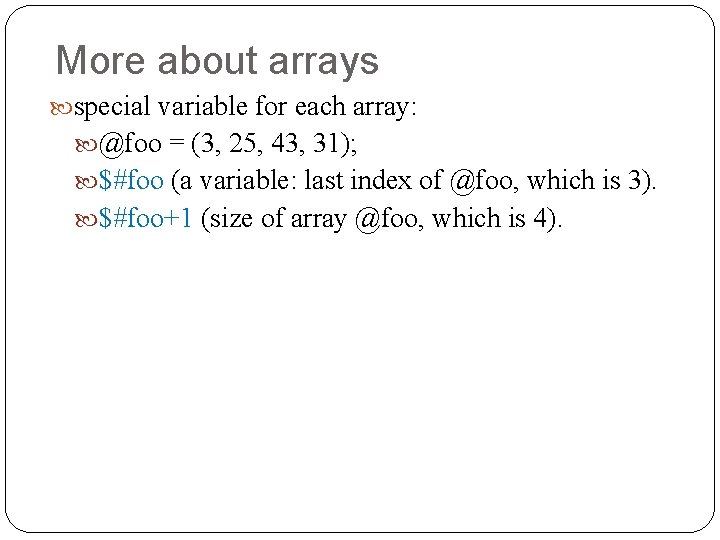More about arrays special variable for each array: @foo = (3, 25, 43, 31); \$#foo (a variable: last index of @foo, which is 3). \$#foo+1 (size of array @foo, which is 4).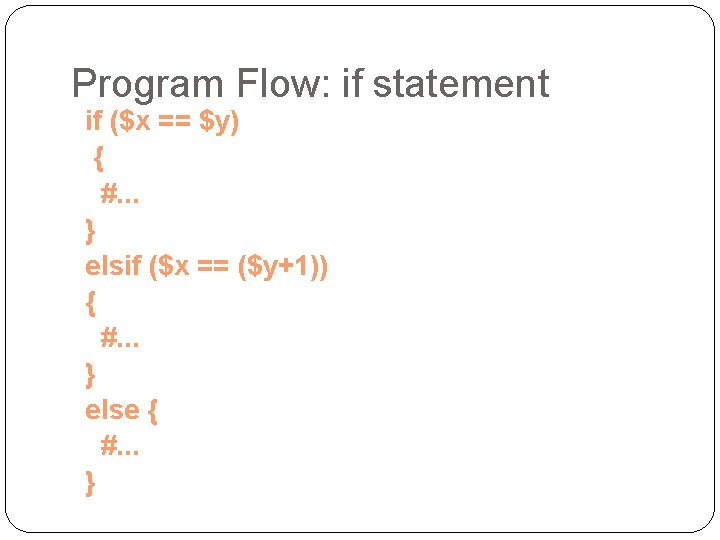Program Flow: if statement if (\$x == \$y) { #. . . } elsif (\$x == (\$y+1)) { #. . . } else { #. . . }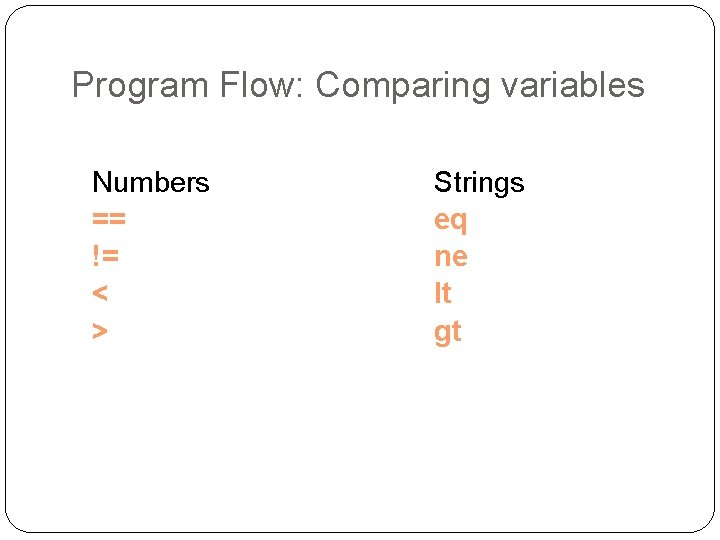Program Flow: Comparing variables Numbers == != < > Strings eq ne lt gtProgram Flow: Logical operators § § § && || ! (logical and) (or) (negation)Program Flow (Loops) for (\$t = 0; \$t < 100; \$t++) { #. . . } while (\$x == \$y) { #. . . }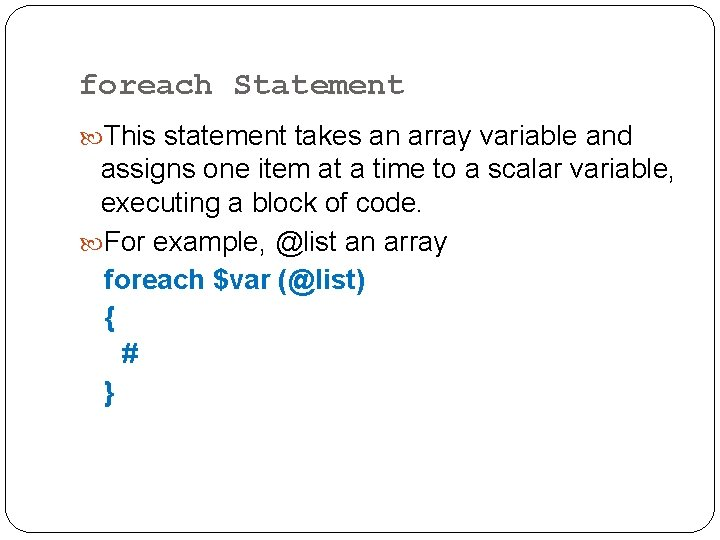foreach Statement This statement takes an array variable and assigns one item at a time to a scalar variable, executing a block of code. For example, @list an array foreach \$var (@list) { # }Basic IO Output to terminal The print statement. Example: print “My name is \$namen”; Input from keyboard The <> operator Example: \$input = <>; read one line from keyboard, and save in variable \$input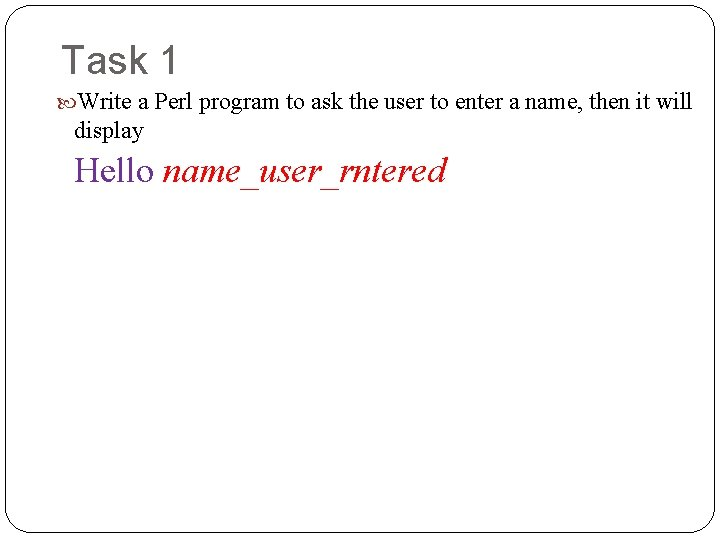Task 1 Write a Perl program to ask the user to enter a name, then it will display Hello name_user_rnteredPerl Program for Task 1 #!/usr/bin/perl print "Enter your name: "; \$name = <>; print "Hello \$namen";Chomp When reading in, carriage return (“n”) is included. Usually don’t want that. chomp will take off the last character of a string, if it is a “n”. chomp (\$foo);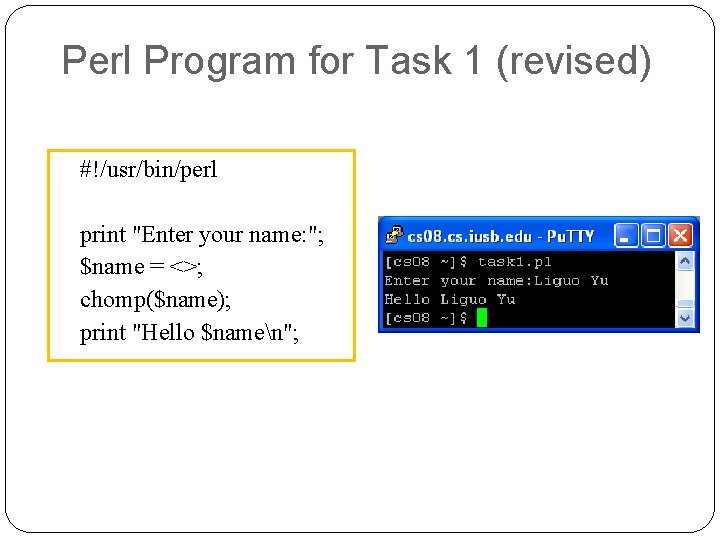Perl Program for Task 1 (revised) #!/usr/bin/perl print "Enter your name: "; \$name = <>; chomp(\$name); print "Hello \$namen";Read / Write to Files To read and write to files we should create something called handles which refer to the files.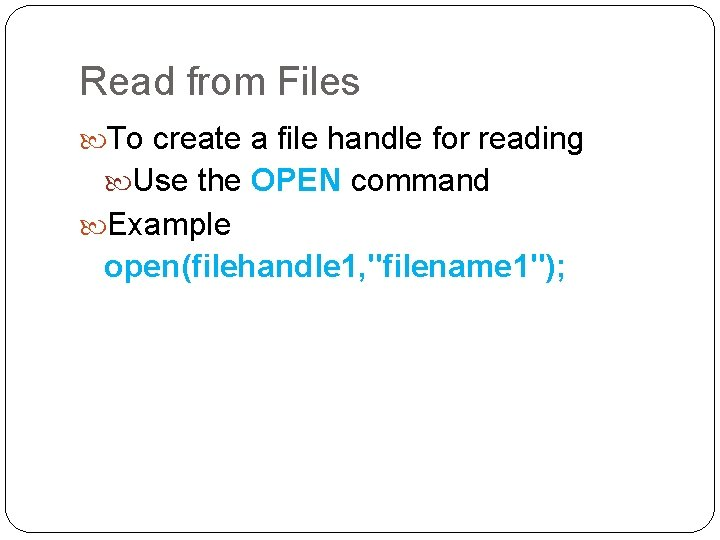Read from Files To create a file handle for reading Use the OPEN command Example open(filehandle 1, "filename 1");Read from Files (continued) Once the file handles have been obtained, we can read data (line by line) from the file. Example: @lines = <filehandle 1>; This will result in each line being read from the file pointed by the file handle and all lines are stored in the array variable @lines, where index 0 (\$lines) contains first line of the file,Read from Files (continued) After read file, we should close the file. close(filehandle 1);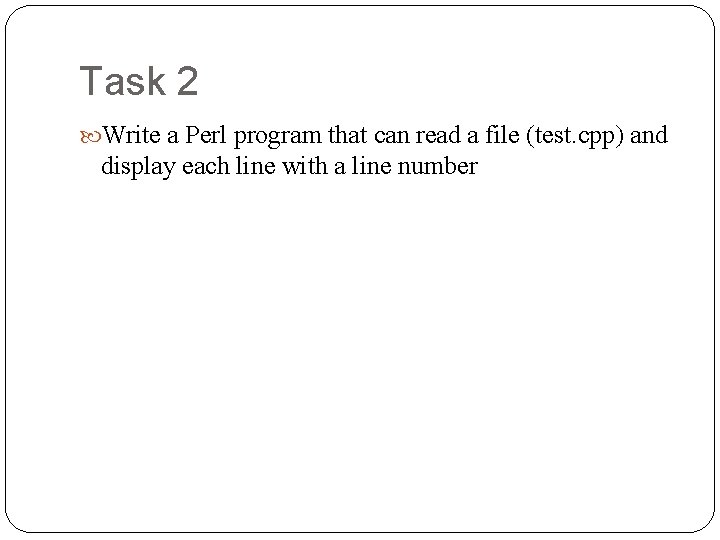Task 2 Write a Perl program that can read a file (test. cpp) and display each line with a line numberPerl program for Task 2 #!/usr/bin/perl open(fh 1, "test. cpp"); @input_lines=<fh 1>; chomp(@input_lines); close(fh 1); \$i=1; foreach \$line(@input_lines) { print "\$i: \$linen"; \$i=\$i+1; }Write to Files To create a file handle for writing Use the OPEN command Example open(filehandle 2, ">filename 2");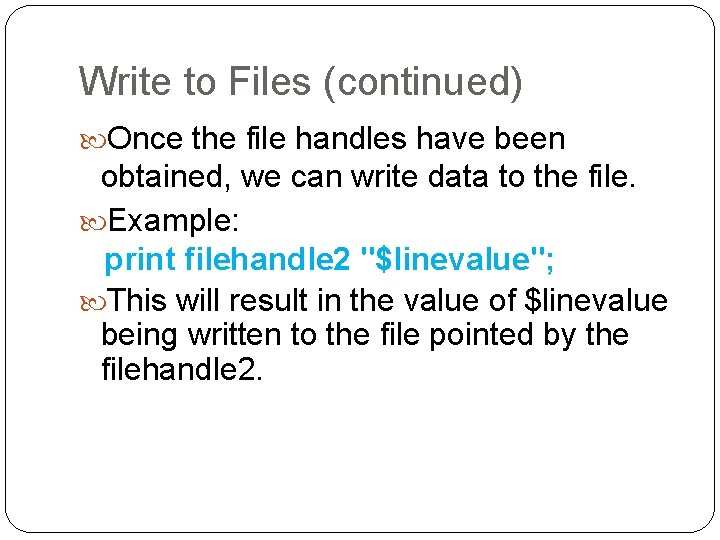Write to Files (continued) Once the file handles have been obtained, we can write data to the file. Example: print filehandle 2 "\$linevalue"; This will result in the value of \$linevalue being written to the file pointed by the filehandle 2.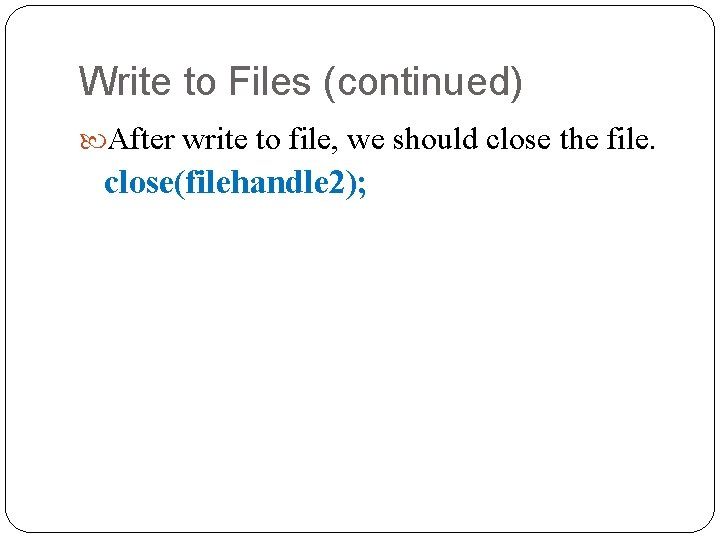Write to Files (continued) After write to file, we should close the file. close(filehandle 2);Task 3 Rewrite the Perl program for Task 2 so that the result will be write to a file (test_c. cpp) instead of displaying on the screen.Perl program for Task 3 #!/usr/bin/perl open(fh 1, "test. cpp"); @input_lines=<fh 1>; chomp(@input_lines); close(fh 1); \$i=1; open(fh 2, ">test_c. cpp"); foreach \$line(@input_lines) { print fh 2 "\$i: \$linen"; \$i=\$i+1; } close(fh 2);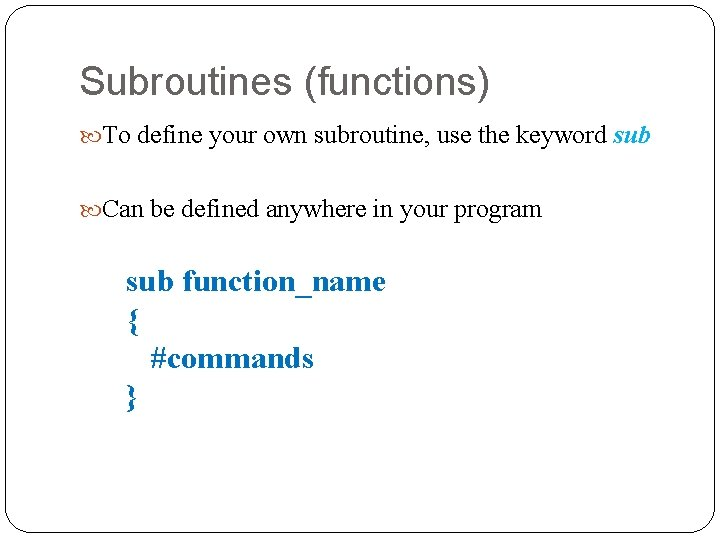Subroutines (functions) To define your own subroutine, use the keyword sub Can be defined anywhere in your program sub function_name { #commands }Function Calls \$Name = getname(); value #return a value #not returning a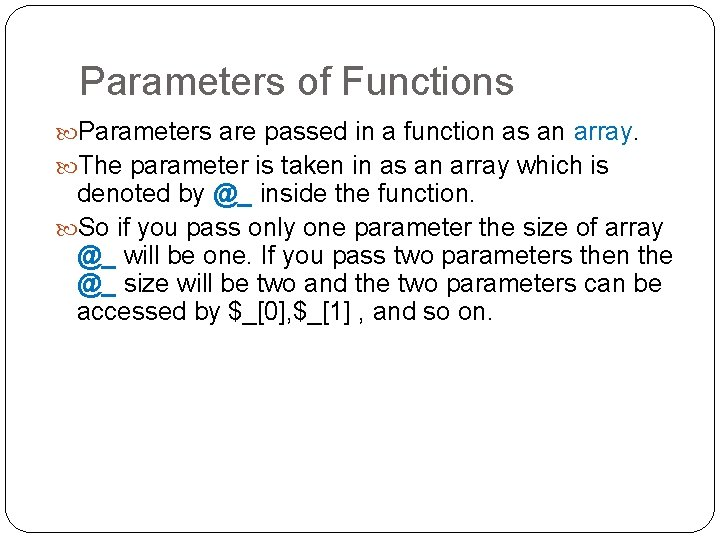Parameters of Functions Parameters are passed in a function as an array. The parameter is taken in as an array which is denoted by @_ inside the function. So if you pass only one parameter the size of array @_ will be one. If you pass two parameters then the @_ size will be two and the two parameters can be accessed by \$_, \$_ , and so on.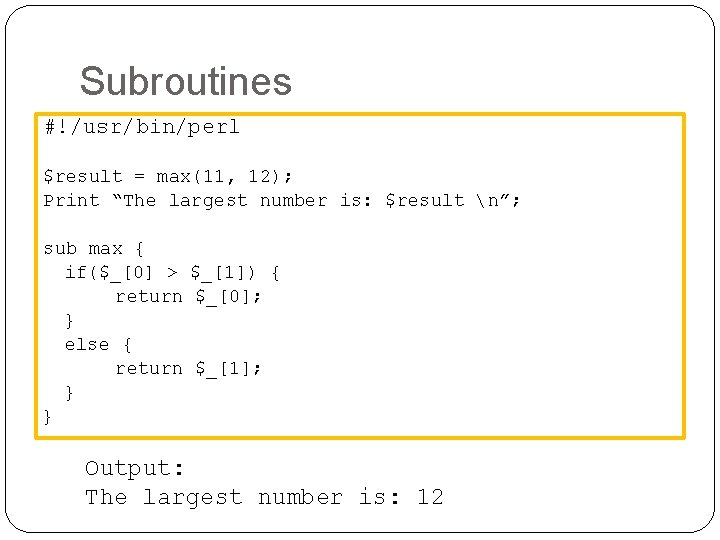Subroutines #!/usr/bin/perl \$result = max(11, 12); Print “The largest number is: \$result n”; sub max { if(\$_ > \$_) { return \$_; } else { return \$_; } } Output: The largest number is: 12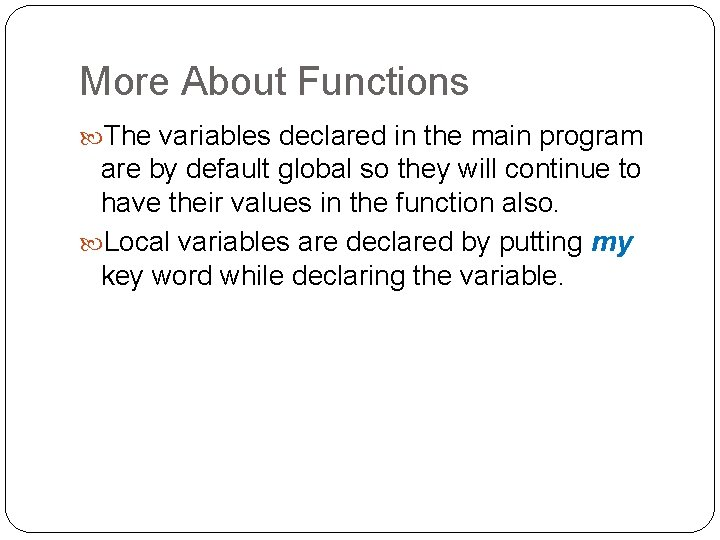More About Functions The variables declared in the main program are by default global so they will continue to have their values in the function also. Local variables are declared by putting my key word while declaring the variable.Subroutines: local variable example #!/usr/bin/perl sub max { my @num = @_ if(\$num > \$num){ return \$num; } else{ return \$num; } } \$result = max(11, 12); Print “The largest number is: \$result n”;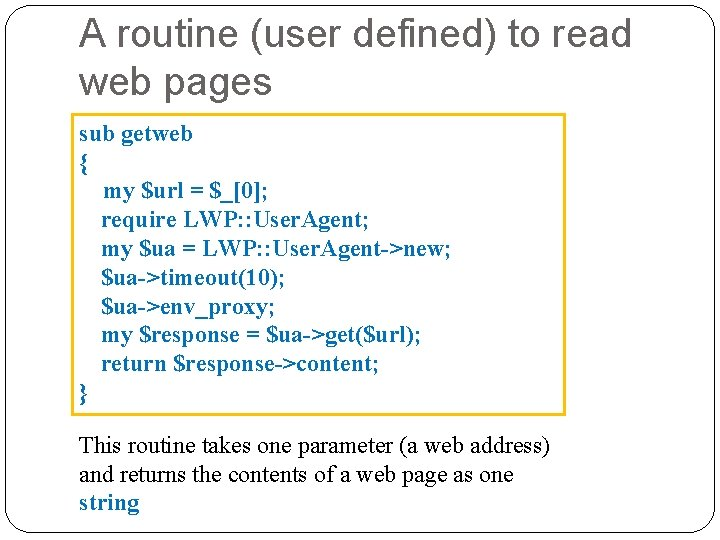A routine (user defined) to read web pages sub getweb { my \$url = \$_; require LWP: : User. Agent; my \$ua = LWP: : User. Agent->new; \$ua->timeout(10); \$ua->env_proxy; my \$response = \$ua->get(\$url); return \$response->content; } This routine takes one parameter (a web address) and returns the contents of a web page as one string